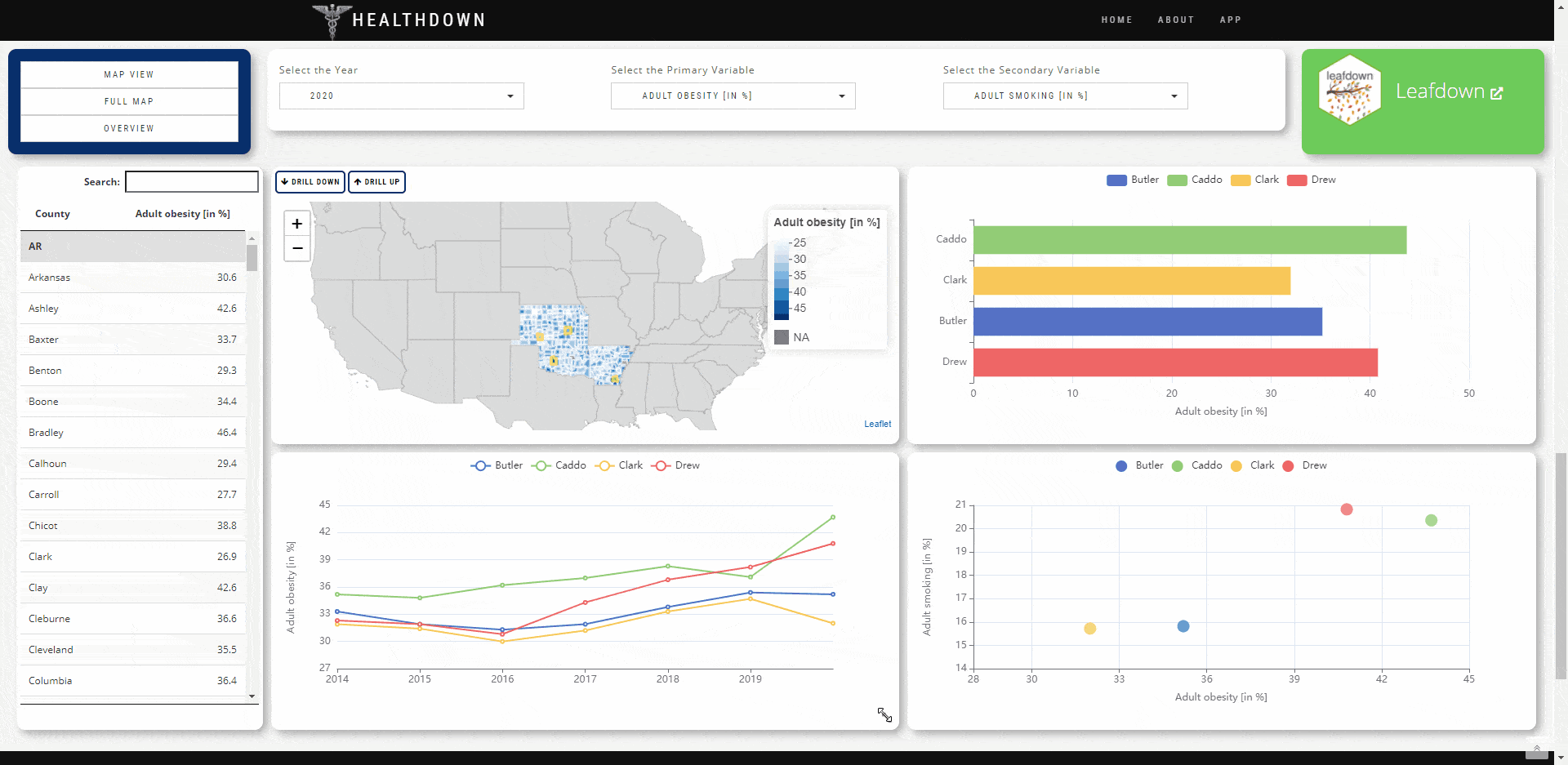# gridstackR

The gridstackR package allows users to easily create Dashboards with gridstack.js functionalities

‘gridstack.js is […] designed to help developers create beautiful draggable, resizable, responsive […] layouts with just a few lines of code’## Installation

You can install the development version from GitHub with:

``````# install.packages("devtools")
devtools::install_github("petergandenberger/gridstackeR")``````

## Example

In the example below we add gridstackR to the basic shiny application ‘Old Faithful Geyser’. The plot can now be dynamically resized and the position for both, the plot and the slider, can be changed using simple drag&drop.

``````library(shiny)
library(gridstackeR)
ui <- fluidPage(
grid_stack(
grid_stack_item(
h = 4, w = 4, id = "plot_container", style = "overflow:hidden",
shinydashboard::box(
title = "Histogram", status = "primary", solidHeader = TRUE,  width = 12, height = "100%",
plotOutput("plot", height = "auto")
)
),
grid_stack_item(
h = 3, w = 4, minH = 3, maxH = 3, id = "slider", style = "overflow:hidden",
shinydashboard::box(
title = "Inputs", status = "warning", solidHeader = TRUE, width = 12, height = "100%",
sliderInput("slider", "Slider input:", 1, 100, 50)
)
)
)
)

server <- function(input, output) {
output\$plot <- renderPlot({
x    <- faithful\$waiting
bins <- seq(min(x), max(x), length.out = input\$slider + 1)

hist(
x, breaks = bins, col = "#75AADB", border = "white",
xlab = "Waiting time to next eruption (in mins)",
main = "Histogram of waiting times"
)
},
# set the height according to the container height (minus the margins)
height = function() {
min_height <- 150
margin <- 80
max(input\$plot_container_height - margin, min_height)
})
}

shinyApp(ui, server)``````

## Usage

In the `ui.R` file, create a grid using `grid_stack(...)` and place grid-stack-items inside using `grid_stack_item(...)`.

Specify options like height, width, x-, y-position as well. Check the gridstack.js documentation for a full list of options.

The `ui.R` file might contain something like the following.

``````grid_stack(
grid_stack_item(
h = 4, w = 4, id = "plot_container", style = "overflow:hidden",
shinydashboard::box(
title = "Histogram", status = "primary", solidHeader = TRUE,  width = 12, height = "100%",
plotOutput("plot", height = "auto")
)
)
)``````

## Dynamic figure height

Elements inside `grid-stack-item` might not change their height automatically.

### Setting the height dynamically using callbacks

The following example shows how the height of the plot can be set dynamically using the `<id>_height` callback

Note: the `plot_container_height` references the height of the `id = "plot_container"` created in the `ui.R` example above.

``````output\$plot <- renderPlot({
x    <- faithful\$waiting
bins <- seq(min(x), max(x), length.out = input\$slider + 1)

hist(
x, breaks = bins, col = "#75AADB", border = "white",
xlab = "Waiting time to next eruption (in mins)",
main = "Histogram of waiting times"
)
},
# set the height according to the container height (minus the margins)
height = function() {
min_height <- 150
margin <- 80
max(input\$plot_container_height - margin, min_height)
}
)``````

### Setting the height for DT::dataTableOutput

The height for a `DT::dataTableOutput` can be set as in the following example.

ui.R

``````grid_stack_item(
w = 4, h = 10, x = 0, y = 0, id =  "c_table",
DT::dataTableOutput("mytable")
)``````

server.R

``````output\$mytable <- DT::renderDataTable({
DT::datatable(mtcars, options = list(
# set the height according to the container height (minus the margins)
scrollY = max(input\$c_table_height, 200) - 110, paging = FALSE
)
)
})``````

### Setting the height for echarts4r

The height for a `echarts4r::echarts4rOutput` can easily be set using the `height="100%"` option.

ui.R

``````grid_stack_item(
w = 5, h = 5, x = 7, y = 0, id = "c_plot",
echarts4rOutput(outputId =  "plot", height = "100%")
)``````

It is also possible to load predefined Layouts. This is demonstrated in the healthdown example above.

ui.R

• every element has to have a unique id
• initialize shinyJS with ‘useShinyjs()’

server.R

• call the function js\$load_grid_stack_layout(layout) with the desired ‘layout’ as a json-array string
• each element of this json-array needs
• the ‘id’ of the grid_stack_item
• the desired new options of this item
``````# do this inside of e.g. an 'observeEvent' of an 'actionButton'
new_layout <- '[
{"id": "c_plot", "options":{"x": 0,"y": 0,"w": 2, "h": 10}},
{"id": "c_map", "options":{"x": 2,"y": 0,"w": 5, "h": 5}}
]'